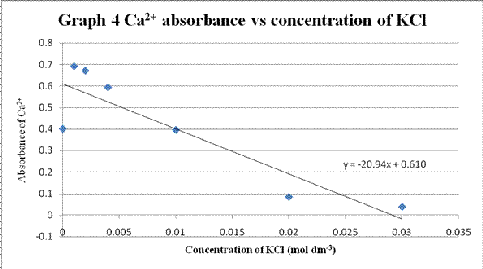## Thursday, July 5, 2012

### Atomic Absorption Spectroscopy (AAS)

Objective:

1. To understand the basic operation the atomic absorption spectroscopy (AAS)

2. To determine the main factors that can affect the absorption’s ability of AAS

Results:

Part A

Table 1 Absorbance of standard solution with different concentration

 Concentration of standard solution of Ca2+ (mg dm-3) Absorbance 1.0 0.045 2.0 0.079 3.0 0.114 4.0 0.148

A graph of Ca2+ absorbance vs concentration of Ca2+ was plotted.

Part B

Table 2 Absorbance of calcium solution when different concentration of aluminium chloride solution added

 Volume of AlCl3 added (cm3) Concentration of Al3+ (g dm-3) Absorbance of Ca2+ 0.0 0.00 1.301 0.5 0.03 0.121 1.0 0.06 0.015 2.0 0.12 0.101 5.0 0.30 0.094 10.0 0.60 0.065 20.0 1.20 0.067

A graph of Ca2+ absorbance vs concentration of Al3+ was plotted (Graph 2).

Part B (II)

Table 3 Absorbance of calcium solution when different concentration of aluminium nitrate solution added

 Volume of Al(NO3)3 added (cm3) Concentration of Al3+ (g dm-3) Absorbance of Ca2+ 0.0 0.00 0.821 0.5 0.01 0.594 1.0 0.02 0.267 2.0 0.04 0.053 5.0 0.10 0.011 10.0 0.20 0.002 20.0 0.40 – 0.001

A graph of Ca2+ absorbance vs concentration of Al3+ was plotted (Graph 3).

Part B (III)

Table 4 Absorbance of calcium solution when different concentration of potassium chloride solution added with the presence of aluminium chloride

 Volume of KCl added (cm3) Concentration of KCl (mol dm-3) Absorbance of Ca2+ 0.0 0.000 0.400 0.5 0.001 0.691 1.0 0.002 0.671 2.0 0.004 0.593 5.0 0.010 0.395 10.0 0.020 0.084 15.0 0.030 0.037

A graph of Ca2+ absorbance vs concentration of KCl was plotted (Graph 4).